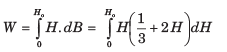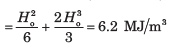Courses

# Test: Magnetostatics

## 20 Questions MCQ Test RRB JE for Electrical Engineering | Test: Magnetostatics

Description
This mock test of Test: Magnetostatics for Railways helps you for every Railways entrance exam. This contains 20 Multiple Choice Questions for Railways Test: Magnetostatics (mcq) to study with solutions a complete question bank. The solved questions answers in this Test: Magnetostatics quiz give you a good mix of easy questions and tough questions. Railways students definitely take this Test: Magnetostatics exercise for a better result in the exam. You can find other Test: Magnetostatics extra questions, long questions & short questions for Railways on EduRev as well by searching above.
QUESTION: 1

### A rectangular loop of wire in free space joins points A(1, 0, 1) to B(3, 0, 1) to C(3, 0, 4) to D(1, 0, 4) to A. The wire carries a current of 6 mA flowing in the uz direction from B to C. A filamentary current of 15 A flows along the entire z, axis in the uz directions. Que: The force on side BC is

Solution: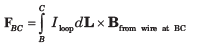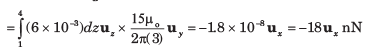QUESTION: 2

### A rectangular loop of wire in free space joins points A(1, 0, 1) to B(3, 0, 1) to C(3, 0, 4) to D(1, 0, 4) to A. The wire carries a current of 6 mA flowing in the uz direction from B to C. A filamentary current of 15 A flows along the entire z, axis in the uz directions. Que: The force on side AB is

Solution:

The field from the long wire now varies with position along the loop segment.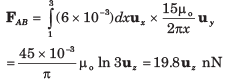QUESTION: 3

### A rectangular loop of wire in free space joins points A(1, 0, 1) to B(3, 0, 1) to C(3, 0, 4) to D(1, 0, 4) to A. The wire carries a current of 6 mA flowing in the uz direction from B to C. A filamentary current of 15 A flows along the entire z, axis in the uz directions. Que: The total force on the loop is

Solution:

This will be the vector sum of the forces on the four sides. By symmetry, the forces on sides AB and CD will be equal and opposite, and so will cancel. This leaves the sum of forces on side BC and DA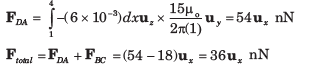QUESTION: 4

Consider the rectangular loop on z = 0 plane shown in fig. The magnetic flux density is B = 6 xux - 9 yuy + 3zuz Wb/m2. The total force experienced by the rectangular loop is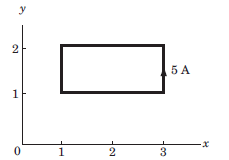Solution: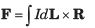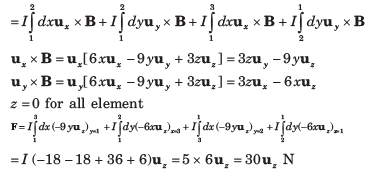QUESTION: 5

Three uniform current sheets are located in free space as follows: 8uz A/m at y = 0, -4uz A/m at y = 1 and -4uz A/m at y = -1. Let F be the vector force per meter length exerted on a current filament carrying 7 mA in the uL direction.

Que: If the current filament is located at x = 0, y = 0.5 andu uL =  uZ , then F is

Solution:

Within the region 1 < y <1, the magnetic fields from the two outer sheets (carrying -4 uz A/m) cancel, leaving only the field from the center sheet. Therefore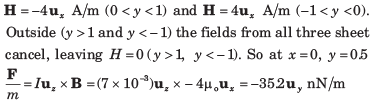QUESTION: 6

Three uniform current sheets are located in free space as follows: 8uz A/m at y = 0, -4uz A/m at y = 1 and -4uz A/m at y = -1. Let F be the vector force per meter length exerted on a current filament carrying 7 mA in the uL direction.

Que: If the current filament is located at y = 0.5, z = 0, and uL = ux, then F is

Solution: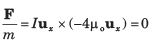QUESTION: 7

Two infinitely long parallel filaments each carry 100 A in the uz direction. If the filaments lie in the plane y = 0 at x = 0 and x = 5 mm, the force on the filament passing through the origin is

Solution: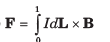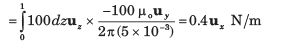QUESTION: 8

A conducting current strip carrying K =  6uz A/m lies in the x = 0 plane between y = 0.5 and y = 1.5 m. There is also a current filament of I = 5 A in the uz direction on the z –axis.

Que: The force exerted on the filament by the current strip is

Solution:

The field from the current strip at the filament location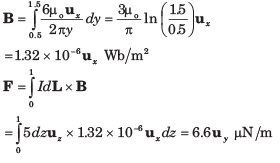QUESTION: 9

A conducting current strip carrying K =  6uz A/m lies in the x = 0 plane between y = 0.5 and y = 1.5 m. There is also a current filament of I = 5 A in the uz direction on the z –axis.

Que: The force exerted on the strip by the filament is

Solution: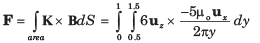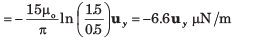QUESTION: 10

In a material the magnetic field intensity is H = 1200A/m when B = 2 Wb/m2. When H is reduced to 400 A/m, B =1.4 Wb/m2. The change in the magnetization M is

Solution: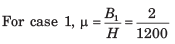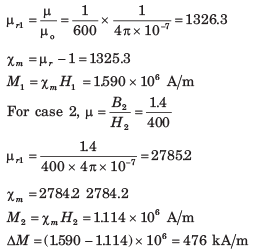QUESTION: 11

In a certain material for which μr = 6.5 ,

H = 10ux + 25uy - 40uz  A/m

Que:

The magnetic susceptibility χm of the material is

Solution: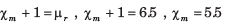QUESTION: 12

In a certain material for which μr = 6.5 , H = 10ux + 25uy - 40uz  A/m

Que: The magnetic flux density B is

Solution: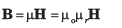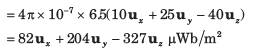QUESTION: 13

In a certain material for which μr = 6.5 , H = 10ux + 25uy - 40uz  A/m

Que: The magnetization M is

Solution: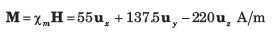QUESTION: 14

In a certain material for which μr = 6.5 , H = 10ux + 25uy - 40uz  A/m

Que: The magnetic energy density is

Solution: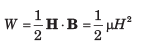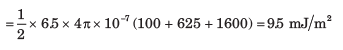QUESTION: 15

For a given material magnetic susceptibility χm = 3.1 and within which B = 0.4 yuz T.

Que: The magnetic field H is

Solution: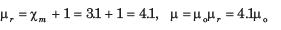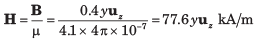QUESTION: 16

For a given material magnetic susceptibility χm = 3.1 and within which B = 0.4 yuz T.

Que: The magnetization M is

Solution: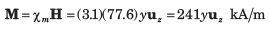QUESTION: 17

A particular material has 2.7 x 1029  atoms/m3 and each atom has a dipole moment of 2.6 x 1030 uy A .m2. The H in material is (μr = 4.2 )

Solution: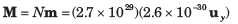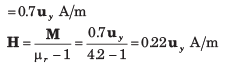QUESTION: 18

In a material magnetic flux density is 0.02 Wb/mand the magnetic susceptibility is 0.003. The magnitude of the magnetization is

Solution: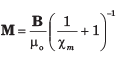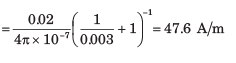QUESTION: 19

A uniform field H = -600 uy A/m exist in free space. The total energy stored in spherical region 1 cm in radius centered at the origin in free space is

Solution: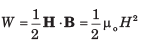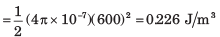QUESTION: 20

The magnetization curve for an iron alloy is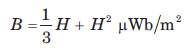approximately given by If H increases from 0 to 210 A/m, the energy stored per unit volume in the alloy is

Solution: